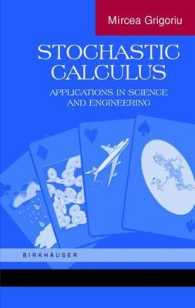### 応用数理科学における確率的問題Stochastic Calculus : Applications in Science and Engineering

• 提携先の海外書籍取次会社に在庫がございます。通常3週間で発送いたします。
重要ご説明事項
1. 納期遅延や、ご入手不能となる場合が若干ございます。
2. 複数冊ご注文の場合、分割発送となる場合がございます。
3. 美品のご指定は承りかねます。
• 【重要：入荷遅延について】
ウクライナ情勢悪化・新型コロナウィルス感染拡大により、洋書・洋古書の入荷が不安定になっています。詳しくはこちらをご確認ください。
海外からのお取り寄せの場合、弊社サイト内で表示している標準的な納期よりもお届けまでに日数がかかる見込みでございます。
申し訳ございませんが、あらかじめご了承くださいますようお願い申し上げます。
• ◆画像の表紙や帯等は実物とは異なる場合があります。
• 製本 Hardcover:ハードカバー版／ページ数 700 p.
• 言語 ENG
• 商品コード 9780817642426
• DDC分類 519.2

Textbook.

### Full Description

Algebraic, differential, and integral equations are used in the applied sciences, en­ gineering, economics, and the social sciences to characterize the current state of a physical, economic, or social system and forecast its evolution in time. Generally, the coefficients of and/or the input to these equations are not precisely known be­ cause of insufficient information, limited understanding of some underlying phe­ nomena, and inherent randonmess. For example, the orientation of the atomic lattice in the grains of a polycrystal varies randomly from grain to grain, the spa­ tial distribution of a phase of a composite material is not known precisely for a particular specimen, bone properties needed to develop reliable artificial joints vary significantly with individual and age, forces acting on a plane from takeoff to landing depend in a complex manner on the environmental conditions and flight pattern, and stock prices and their evolution in time depend on a large number of factors that cannot be described by deterministic models. Problems that can be defined by algebraic, differential, and integral equations with random coefficients and/or input are referred to as stochastic problems. The main objective of this book is the solution of stochastic problems, that is, the determination of the probability law, moments, and/or other probabilistic properties of the state of a physical, economic, or social system. It is assumed that the operators and inputs defining a stochastic problem are specified.

### Contents

Introduction * Probability Theory * Stochastic Processes * Itô's Formula and Stochastic Differential Equations * Monte Carlo Simulation * Deterministic System and Input * Deterministic System and Stochastic Input * Stochastic System and Deterministic Input * Stochastic System and Stochastic Input * Bibliography * Index